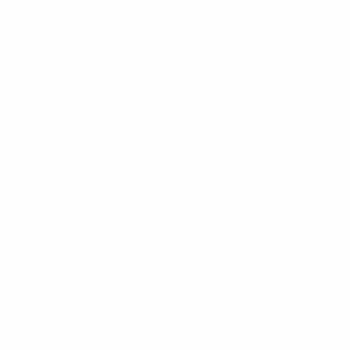Sorry, you do not have permission to ask a question, You must login to ask a question.

# NPTEL Introduction to Machine Learning Assignment Answers Week 4 2022 IITKGP

Are you looking for help in Machine Learning NPTEL Week 4 Assignment Answers? So, here in this article, we have provided Machine Learning week 4 Assignment Answer’s hint.

## NPTEL Introduction to Machine Learning Assignment Answers Week 4

Q1. A man is known to speak the truth 2 out of 3 times. He throws a die and reports that the number obtained is 4. Find the probability that the number obtained is actually 4:

a. 2/3
b. 3/4
c. 5/22
d. 2/7

Q2. Consider the following graphical model, mark which of the following pair of random variables are independent given no evidence?

A. a,b

B. c,d

C. e,d

D. c,e

Q3. Two cards are drawn at random from a deck of 52 cards without replacement. What is the probability of drawing a 2 and an Ace in that order?

a. 4/51

b. 1/13

c. 4/256

d. 4/663

Q4. Consider the following Bayesian network. The random variables given in the model are modeled as discrete variables (Rain = R, Sprinkler = S and Wet Grass = W) and the corresponding probability values are given below.

P(R) = 0.1

P(S) = 0.2

P(WR, S) = 0.8

P(WIR,S)= 0.7

P(WR, S) = 0.6

P(WR,S) = 0.5

Calculate P(S| W, R).

a. 1.

b. 0.5.

c. 0.22.

d. 0.78

Q5. What is the naive assumption in a Naive Bayes Classifier?

a. All the classes are independent of each other

b. All the features of a class are independent of each other

c. The most probable feature for a class is the most important feature to be considered for classification

d. All the features of a class are conditionally dependent on each other.

Answer: b. All the features of a class are independent of each other

Q6. A drug test (random variable T) has 1% false positives (ie., 1% of those not taking drugs show positive in the test), and 5% false negatives (i.e., 5% of those taking drugs test negative). Suppose that 2% of those tested are taking drugs. Determine the probability that somebody who tests positive is actually taking drugs (random variable D).

a. 0.66

b. 0.34

c. 0.50

d. 0.91

Q7. It is given that P(A|B) = 2/3 and P(A|B) = 1/4. Compute the value of P(B|A).

a. 1/2

b. 2/3

c. 3/4

d.  Not enough information.

Q8. What is the joint probability distribution in terms of conditional probabilities?

a. P(D1) * P(D2|D1) * P(S1|D1) * P(S2|D1) * P(S3|D2)

b. P(D1) * P(D2) * P(S1|D1) * P(S2|D1) * P(S3|D1, D2)

c. P(D1) * P(D2) * P(S1|D2) * P(S2|D2) * P(S3|D2)

d. P(D1) * P(D2) * P(S1|D1) * P(S2|D1, D2) * P(S3|D2)

Answer: d. P(D1) * P(D2) * P(S1|D1) * P(S2|D1, D2) * P(S3|D2)

Q9. Suppose P(D1)=0.5. P(D2)=0.6. P(S1 D1)=0.4 and P(S1| D1′)=0.6. Find P(S1)

a. 0.14

b. 0.36

c. 0.50

d. 0.66

Q10. In a Bayesian network a node with only outgoing edge(s) represents

a. a variable conditionally independent of the other variables.

b. a variable dependent on its siblings.

c. a variable whose dependency is uncertain.

d. None of the above.

Answer: a. a variable conditionally independent of the other variables.

Disclaimer: These answers are provided only for the purpose to help students to take references. This website does not claim any surety of 100% correct answers. So, this website urges you to complete your assignment yourself.

Also Available:

NPTEL Introduction to Machine Learning Assignment Answers Week 3

NPTEL Introduction to Machine Learning Assignment Answers Week 5

## NPTEL Cloud Computing Assignment 5 Answers 2023